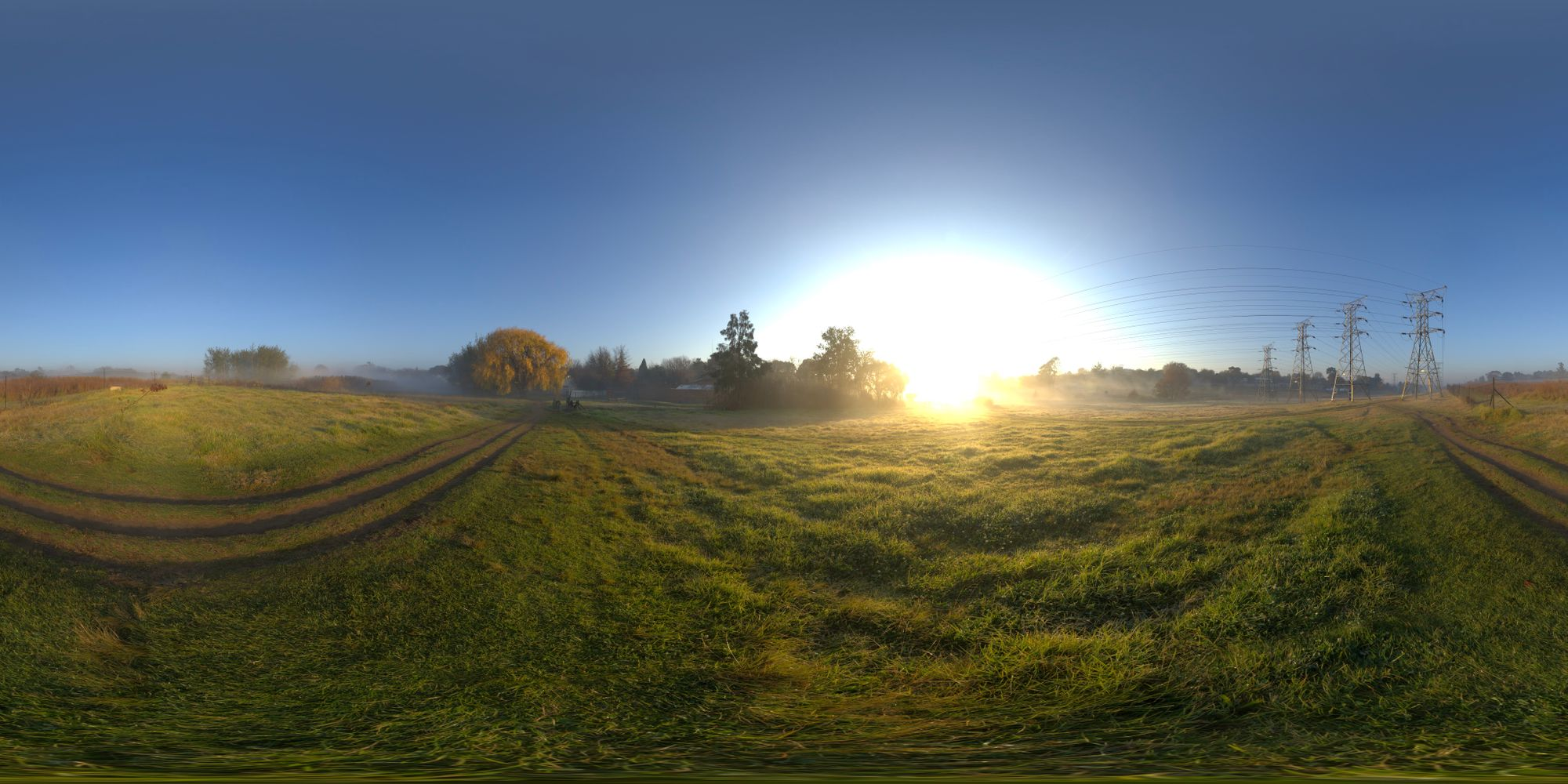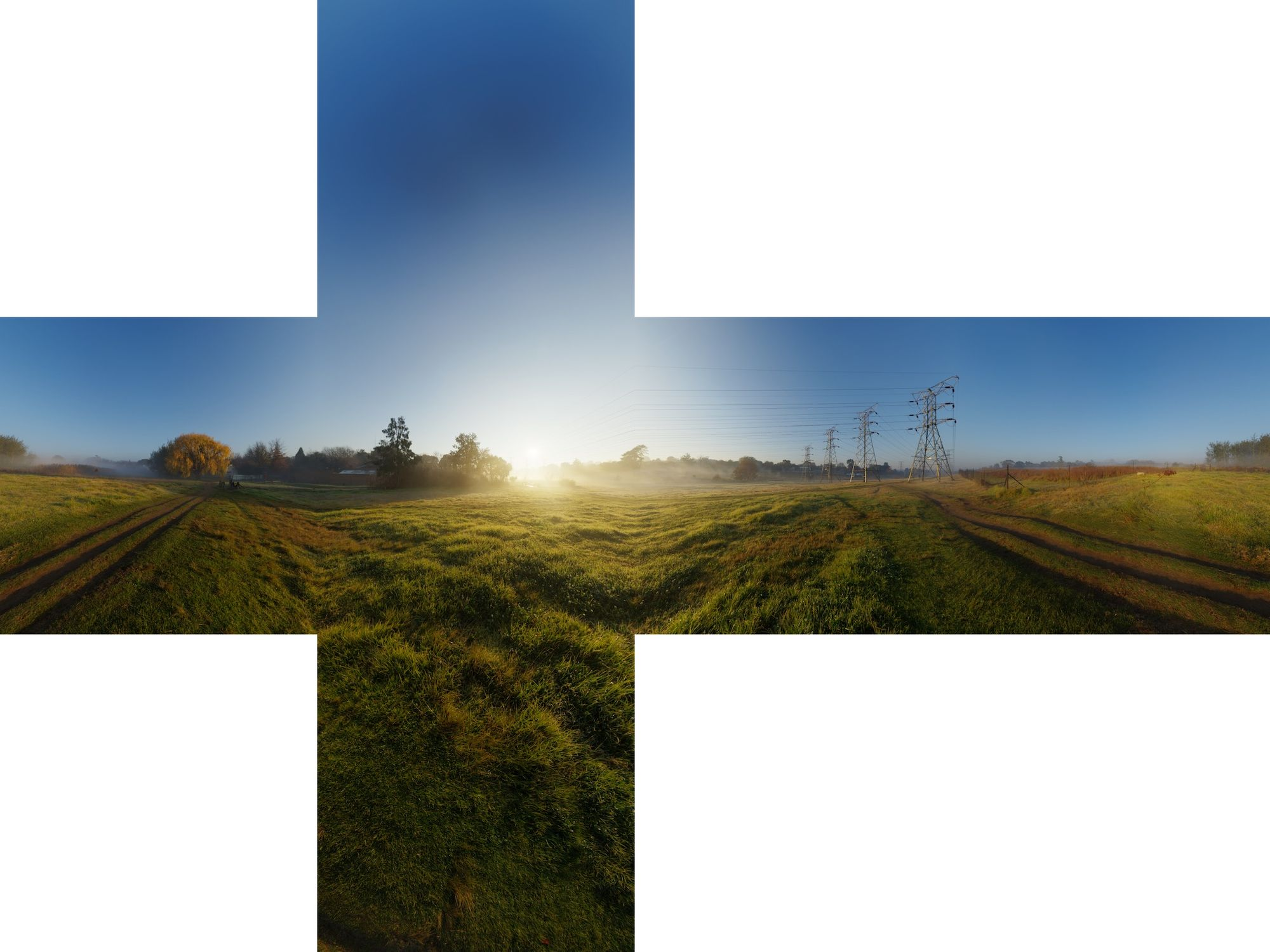In this post I want you to show how to load an Panorama Image into an Cube Map. The Panorama Image is commonly abbreviated as HDRI. It is often an HDRI, but the hard part is not loading an HDRI but changing the Panorama-Texture to an CubeMap-Texture.

### Intro

If you want to know more about Cubemaps I can recommend the Tutorial at Learnopengl.com. And of course more about HDRIs in general: https://en.wikipedia.org/wiki/High_Dynamic_Range_(photography_technique)

and this Wikipedia Article about Cube Mapping: https://en.wikipedia.org/wiki/Cube_mapping

Basically we have a Panorama Image, which looks like this:Panorama Image - which you can find for example on HdriHavenThis is what we want as a Texture for all our cube sides.

And we need to turn this Panorama into six textures.

Above you see a Cubemap Texture where each individual face is on one big texture. If you are beginning with skyboxes I recommend you look at the tutorial at learnopengl.com and try to load a texture like the one above. You can create those textures at: https://matheowis.github.io/HDRI-to-CubeMap/.  They are not HDRIs, but for testing your Skybox in your engine its a really great tool.

Now that we know what we have to do we can imagine a cube in a 3D-World and each face of the cube is a camera. The view angle of that camera has to be 90 degrees, so that everything gets covered.

For a better understanding I made a little Sketch which describes the situation out of a 2d perspective.

The circle around should be the HDRI Sphere, on that Sphere the HDRI Panorama is mapped. As you can see the 6 cameras really cover everything.

To make this in OpenGl happen you could make up a scene rotate the camera for each face and render that to a framebuffer, use that render output for a CubeTexture. And basically it is exactly what we will do.  I looked at the Gltf Example Viewer from Khronos, and how they did it, and they did it really nice. https://github.com/KhronosGroup/glTF-Sample-Viewer

They create the Skybox-Cubemap in these basic steps:

1. Create an HDRI Input Texture (The Texture where your Panorama image gets loaded into)
2. Create the Cube Map Texture - The Cube Texture used by your Skybox shader.
3. Create a Framebuffer to render into.
5. Convert the Panorama to the Cubemap

I will now talk a bit about each single step.

### Create your HDRI Input Texture

Create your Texture how you would normally do this like this:

``````glActiveTexture(GL_TEXTURE0 + texture_unit);
glGenTextures(1, &gl_texture_name);
glBindTexture(gl_target, gl_texture_name);
``````

Upload the image data to the GPU:

``````upload_image_data(img, this->gl_target, is_in_srgb);
``````

set your Texture Parameters and MipMap generation:

``````glTexParameteri(gl_target, GL_TEXTURE_WRAP_S, gl_wrap_s);
glTexParameteri(gl_target, GL_TEXTURE_WRAP_T, gl_wrap_t);

glTexParameteri(gl_target, GL_TEXTURE_MIN_FILTER, gl_filter_min);
glTexParameteri(gl_target, GL_TEXTURE_MAG_FILTER, gl_filter_mag);

glGenerateMipmap(gl_target);
``````

``````    [[maybe_unused]] inline static void upload_image_data(Image &img, GLenum target = GL_TEXTURE_2D, bool is_in_srgb = false) {
GLenum internal_format = 0x00;
GLenum byte_format;

std::string debug_msg{};
std::string debug_format{};

switch(img.channels) {

//TODO CASE 1

case 3: {
switch (img.bits) {
case 0:
case 8: {
if(is_in_srgb) {
internal_format = GL_SRGB8;
} else {
internal_format = GL_RGB8;
}
byte_format = GL_UNSIGNED_BYTE;
} break;
case 16: {
internal_format = GL_RGB16;
byte_format = GL_UNSIGNED_SHORT;
} break;
case 32: {
internal_format = GL_RGB32F;
byte_format = GL_FLOAT;
} break;
default:
throw std::logic_error("image format not supported");
}

debug_format = "RGB";
assert(internal_format);

glTexImage2D(target, 0, internal_format,
img.width, img.height,
0, GL_RGB, byte_format, img.data);
} break;

case 4: {
switch (img.bits) {
case 0:
case 8: {
internal_format = GL_RGBA8;
if(is_in_srgb) {
internal_format = GL_SRGB8_ALPHA8;
} else {
internal_format = GL_RGB8;
}

byte_format = GL_UNSIGNED_BYTE;
} break;
case 16: {
internal_format = GL_RGBA16;
byte_format = GL_UNSIGNED_SHORT;
} break;
case 32: {
internal_format = GL_RGBA32F;
byte_format = GL_FLOAT;
} break;
default:
throw std::logic_error("image format not supported");
}

debug_format = "RGBA";

glTexImage2D(target, 0, internal_format,
img.width, img.height,
0, GL_RGBA, byte_format, img.data);
} break;

default: {
assert(0);
}
}

spdlog::debug("[OpenGl] image upload complete {}: {} x {}, {}-bit-color {}",
img.name,
img.width, img.height,
img.bits, debug_format);

}
``````

If you are loading your images with stb_image you have to use another function for loading hdri because of the 32bit color information. This is how I do this:

``````    void PngLoader::load_png(Image &png_image,
const std::string &filepath_str, bool vert_flip, bool linear_f) {

int w = 0;
int h = 0;
int channels = 0;

if(!linear_f) { //no float data
png_image.data = stbi_load(filepath_str.c_str(), &w, &h, &channels, 0);
} else { //float data like hdr images
png_image.data = reinterpret_cast<const unsigned char *>(stbi_loadf(filepath_str.c_str(), &w, &h, &channels,
0));
png_image.bits = 8 * sizeof(float);
}

if(png_image.data == nullptr) {
}

png_image.width = w;
png_image.height = h;
png_image.channels = channels;

}
``````

### Create the CubeMap Texture

Creating a Cubemap Texture you have to call `glTexImage2D` six times.

``````    void Texture::create_cubemap(bool with_mipmaps) {

assert(initalized);
assert(this->gl_target == GL_TEXTURE_CUBE_MAP);

bind();

for(int i = 0; i < 6; ++i) {
reset_gl_error();
auto data = std::vector<unsigned char>();
glTexImage2D(GL_TEXTURE_CUBE_MAP_POSITIVE_X + i,
0, GL_RGBA32F, this->width, this->height,
0, GL_RGBA, GL_FLOAT, nullptr);
assert_gl_error();
}

gl_filter_min = (with_mipmaps) ? GL_LINEAR_MIPMAP_LINEAR : GL_LINEAR;
gl_filter_mag = GL_LINEAR;

gl_wrap_r = GL_CLAMP_TO_EDGE;
gl_wrap_s = GL_CLAMP_TO_EDGE;
gl_wrap_t = GL_CLAMP_TO_EDGE;

glTexParameteri(gl_target, GL_TEXTURE_MIN_FILTER, gl_filter_min);
glTexParameteri(gl_target, GL_TEXTURE_MAG_FILTER, gl_filter_mag);

glTexParameteri(gl_target, GL_TEXTURE_WRAP_R, gl_wrap_r);
glTexParameteri(gl_target, GL_TEXTURE_WRAP_S, gl_wrap_s);
glTexParameteri(gl_target, GL_TEXTURE_WRAP_T, gl_wrap_t);

spdlog::debug("[Texture][{}] created empty {} [{}:{}]", name, magic_enum::enum_name(this->m_type), width, height);
initalized = true;
}
``````

### Convert the Panorama to the CubeMap

And here is my Panorama-to-CubeMap, which is basically the same as from https://github.com/KhronosGroup/glTF-Sample-Viewer with some changes.

``````
void IblSampler::panorama_to_cubemap() {
spdlog::debug("[IblSampler] panorama_to_cubemap()");
assert(cubemap_texture.isInitalized());

glBindVertexArray(1);

for(int i = 0; i < 6; ++i) {

framebuffer.bind();
int side = i;
reset_gl_error();
glFramebufferTexture2D(GL_FRAMEBUFFER,
GL_COLOR_ATTACHMENT0,
GL_TEXTURE_CUBE_MAP_POSITIVE_X + side,
cubemap_texture.getGlTexture(),
0);

assert_gl_error();

cubemap_texture.bind();

glViewport(0, 0, texture_size, texture_size);
glClearColor(0.5f, 0.5f, 0.5f, 0.f);
glClear(GL_COLOR_BUFFER_BIT | GL_DEPTH_BUFFER_BIT);
assert_gl_error();
assert_gl_error();

//input_texture.bind();
glActiveTexture(GL_TEXTURE0 + input_texture.getTextureUnit());
glBindTexture(input_texture.getGlTarget(), input_texture.getGlTexture());
assert_gl_error();

assert_gl_error();

glDrawArrays(GL_TRIANGLES, 0, 3);
assert_gl_error();
}

cubemap_texture.bind();
cubemap_texture.generate_mipmap();

}
``````

Basically we call `glFramebufferTexture2D` for each side. The interesing parts are happening in the shaders. These are 1:1 the same shaders as from https://github.com/KhronosGroup/glTF-Sample-Viewer

``````precision highp float;

out vec2 texCoord;

void main(void)
{
float x = float((gl_VertexID & 1) << 2);
float y = float((gl_VertexID & 2) << 1);
texCoord.x = x * 0.5;
texCoord.y = y * 0.5;
gl_Position = vec4(x - 1.0, y - 1.0, 0, 1);
}
``````

For the Vertex-Shader they use a little trick where you don't need to upload vertex data. More about that nice trick here: https://rauwendaal.net/2014/06/14/rendering-a-screen-covering-triangle-in-opengl/

We will go through this step by step:

``````vec3 uvToXYZ(int face, vec2 uv)
{
if(face == 0)
return vec3(     1.f,   uv.y,    -uv.x);

else if(face == 1)
return vec3(    -1.f,   uv.y,     uv.x);

else if(face == 2)
return vec3(   +uv.x,   -1.f,    +uv.y);

else if(face == 3)
return vec3(   +uv.x,    1.f,    -uv.y);

else if(face == 4)
return vec3(   +uv.x,   uv.y,      1.f);

else //if(face == 5)
{	return vec3(    -uv.x,  +uv.y,     -1.f);}
}
``````

This is actually almost the same you can find on Wikipedia. With that coordinates you can calculate the uv coordinates on the "sphere" panorama image.

``````vec2 dirToUV(vec3 dir)
{
return vec2(
0.5f + 0.5f * atan(dir.z, dir.x) / MATH_PI,
1.f - acos(dir.y) / MATH_PI);
}
``````

Alltogehter:

``````vec3 panoramaToCubeMap(int face, vec2 texCoord)
{
vec2 texCoordNew = texCoord*2.0-1.0; //< mapping vom 0,1 to -1,1 coords
vec3 scan = uvToXYZ(face, texCoordNew);
vec3 direction = normalize(scan);
vec2 src = dirToUV(direction);

return  texture(u_panorama, src).rgb; //< get the color from the panorama
}
``````